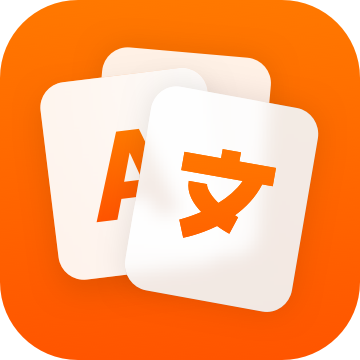By using this site, you agree that we're using cookies to save preferences and for telemetry data such as Google Analytics.

Indicative

Present Simple
Perfect Simple
Past Simple
Pluperfect Simple
Future Simple
Future perfect Simple
Present Continuous
Perfect Continuous
Past Continuous
Pluperfect Continuous
Future Continuous
Future perfect Continuous
 I astipulate you astipulate he/she/it astipulates we astipulate they astipulate
 I have astipulated you have astipulated he/she/it has astipulated we have astipulated they have astipulated
 I astipulated you astipulated he/she/it astipulated we astipulated they astipulated
 I will astipulate you will astipulate he/she/it will astipulate we will astipulate they will astipulate
 I will have astipulated you will have astipulated he/she/it will have astipulated we will have astipulated they will have astipulated
 I am astipulating you are astipulating he/she/it is astipulating we are astipulating they are astipulating
 I have been astipulating you have been astipulating he/she/it has been astipulating we have been astipulating they have been astipulating
 I was astipulating you were astipulating he/she/it was astipulating we were astipulating they were astipulating
 I had been astipulating you had been astipulating he/she/it had been astipulating we had been astipulating they had been astipulating
 I will be astipulating you will be astipulating he/she/it will be astipulating we will be astipulating they will be astipulating
 I will have been astipulating you will have been astipulating he/she/it will have been astipulating we will have been astipulating they will have been astipulating

Subjunctive

Present Simple
Perfect Simple
Imperfect Simple
Pluperfect Simple
 I astipulate you astipulate he/she/it astipulate we astipulate they astipulate
 I have astipulated you have astipulated he/she/it have astipulated we have astipulated they have astipulated
 I astipulated you astipulated he/she/it astipulated we astipulated they astipulated

Conditional

Present Simple
Perfect Simple
Present Continuous
Perfect Continuous
 I would astipulate you would astipulate he/she/it would astipulate we would astipulate they would astipulate
 I would have astipulated you would have astipulated he/she/it would have astipulated we would have astipulated they would have astipulated
 I would be astipulating you would be astipulating he/she/it would be astipulating we would be astipulating they would be astipulating
 I would have been astipulating you would have been astipulating he/she/it would have been astipulating we would have been astipulating they would have been astipulating

Imperative

 you astipulate we Let's astipulate

← Conjugate another English verbLearn languages with Reji.

One-time purchase for a reasonable price.
No subscriptions, no hidden costs.